# SVM

## 1. 基本概念

• 硬间隔支持向量机（线性可分支持向量机）：当训练数据线性可分时，可通过硬间隔最大化学得一个线性可分支持向量机。
• 软间隔支持向量机：当训练数据近似线性可分时，可通过软间隔最大化学得一个线性支持向量机。
• 非线性支持向量机：当训练数据线性不可分时，可通过核方法以及软间隔最大化学得一个非线性支持向量机。

## 2. 硬间隔支持向量机

w⃗ Tx⃗ +b=0(1) (1) w → T x → + b = 0

f(x⃗ )=sign(wx⃗ +b)(2) (2) f ( x → ) = s i g n ( w ∗ → x → + b ∗ )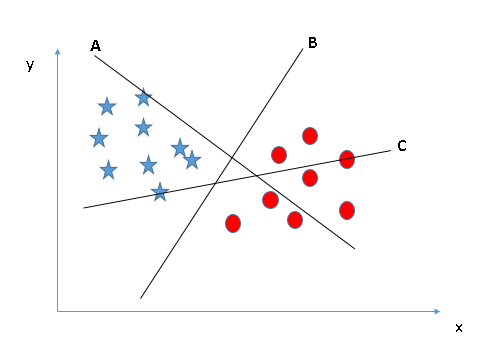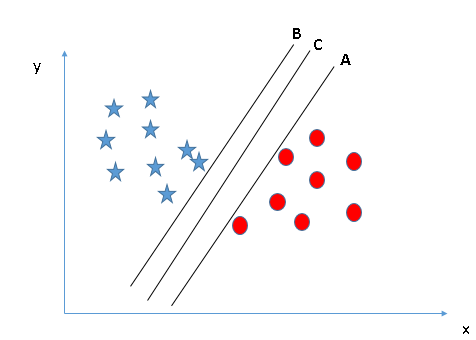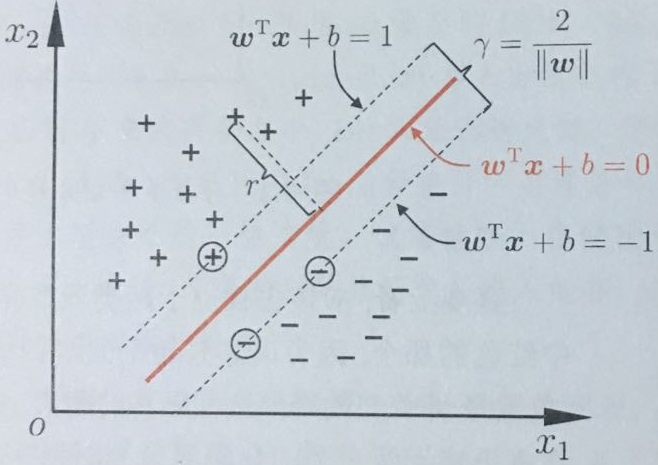r=w⃗ x⃗ +b||w⃗ ||(3) (3) r = w → x → + b | | w → | |

{w⃗ Txi+b+1,w⃗ Txi+b1,yi=+1yi=1(4) (4) { w → T x i → + b ≥ + 1 , y i = + 1 w → T x i → + b ≤ − 1 , y i = − 1

yi(w⃗ Txi+b)+1(5) (5) y i ( w → T x i → + b ) ≥ + 1

γ=(x+x)w⃗ ||w⃗ ||=x+w⃗ ||w⃗ ||xw⃗ ||w⃗ ||(6) (6) γ = ( x + → − x − → ) w → | | w → | | = x + → w → | | w → | | − x − → w → | | w → | |
w⃗ x++b=+1 w → x + → + b = + 1 $\vec{w}\vec{x_+}+b=+1$ w⃗ x+b=1 w → x − → + b = − 1 $\vec{w}\vec{x_-}+b=-1$，即：
{w⃗ x+=1bw⃗ x+=1b(7) (7) { w → x + → = 1 − b w → x + → = − 1 − b

γ=2||w⃗ ||(8) (8) γ = 2 | | w → | |

{maxw⃗ ,b2||w⃗ ||s.t.yi(w⃗ Txi+b)+1,i=1,2,,n(9) (9) { max w → , b 2 | | w → | | s . t . y i ( w → T x i → + b ) ≥ + 1 , i = 1 , 2 , … , n

{minw⃗ ,b12||w⃗ ||2s.t.yi(w⃗ Txi+b)+1,i=1,2,,n(10) (10) { min w → , b 1 2 | | w → | | 2 s . t . y i ( w → T x i → + b ) ≥ + 1 , i = 1 , 2 , … , n

### 2.1 拉格朗日对偶问题

$\begin{array}{}\text{(11)}& f\left(\stackrel{\to }{x}\right)=sign\left(\stackrel{\to }{w}\stackrel{\to }{x}+b\right)\end{array}$

L(w⃗ ,b,α⃗ )=12||w⃗ ||2i=1nαi(yi(w⃗ Txi+b)1)(12) (12) L ( w → , b , α → ) = 1 2 | | w → | | 2 − ∑ i = 1 n α i ( y i ( w → T x i + b ) − 1 )

L(w⃗ ,b,α⃗ ) L ( w → , b , α → ) $L(\vec{w},b,\vec{\alpha})$ w⃗  w → $\vec{w}$ b b $b$求偏导并将偏导数等于零可得：
$\begin{array}{}\text{(13)}& \left\{\begin{array}{l}\stackrel{\to }{w}=\sum _{i=1}^{n}{\alpha }_{i}{y}_{i}\stackrel{\to }{{x}_{i}}\\ \sum _{i=1}^{n}{\alpha }_{i}{y}_{i}=0\end{array}\end{array}$

$\begin{array}{}\text{(14)}& \left\{\begin{array}{l}\underset{\alpha }{max}\sum _{i=1}^{n}{\alpha }_{i}-\frac{1}{2}\sum _{i=1}^{n}\sum _{j=1}^{n}{\alpha }_{i}{\alpha }_{j}{y}_{i}{y}_{j}{\stackrel{\to }{{x}_{i}}}^{T}\stackrel{\to }{{x}_{j}}\\ s.t.\phantom{\rule{1em}{0ex}}{\alpha }_{i}\ge 0,i=1,2,\dots ,n\\ \phantom{\rule{1em}{0ex}}\phantom{\rule{1em}{0ex}}\phantom{\rule{1em}{0ex}}\sum _{i=1}^{n}{\alpha }_{i}{y}_{i}=0\end{array}\end{array}$

$\begin{array}{}\text{(15)}& f\left(\stackrel{\to }{x}\right)={\stackrel{\to }{w}}^{T}\stackrel{\to }{x}+b=\sum _{i=1}^{n}{\alpha }_{i}{y}_{i}{\stackrel{\to }{{x}_{i}}}^{T}\stackrel{\to }{x}+b\end{array}$

### 2.2 SVM问题的KKT条件

αi0yi(w⃗ Tx⃗ +b)10,i=1,2,,nαi(yi(w⃗ Tx⃗ +b)1)=0(16) (16) { α i ≥ 0 y i ( w → T x → + b ) − 1 ≥ 0 , i = 1 , 2 , … , n α i ( y i ( w → T x → + b ) − 1 ) = 0

{w⃗ =ni=1αiyixiyi(w⃗ Tx⃗ +b)1=0(17) (17) { w → = ∑ i = 1 n α i y i x i → y i ( w → T x → + b ) − 1 = 0

$\begin{array}{}\text{(18)}& f\left(\stackrel{\to }{x}\right)=sign\left({\stackrel{\to }{w}}^{T}\stackrel{\to }{x}+b\right)\end{array}$

## 3. 软间隔支持向量机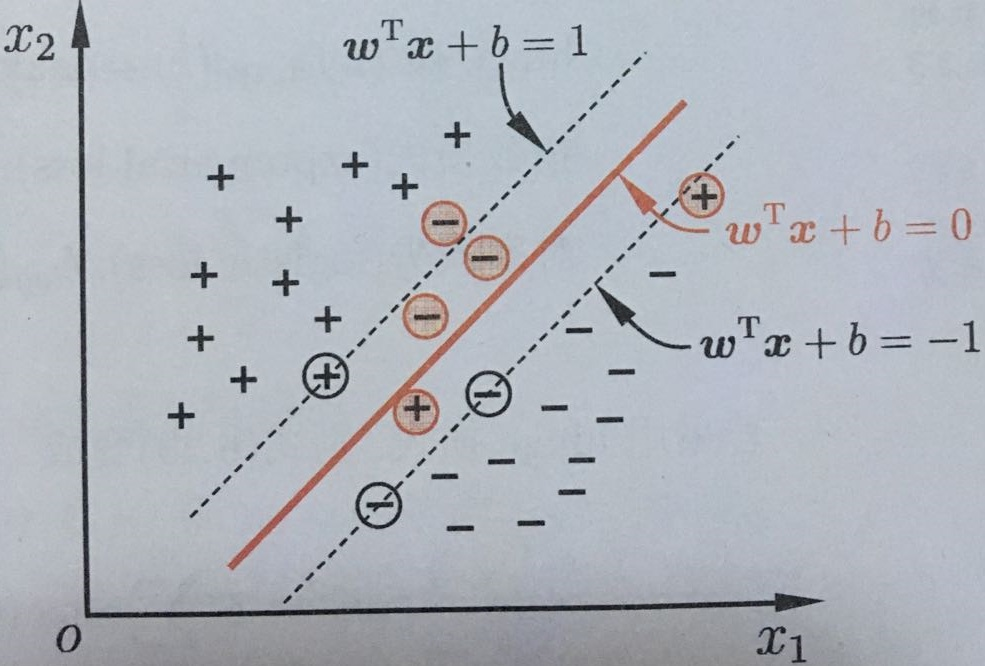minw⃗ ,b(12||w⃗ ||2+Cni=1ξi)s.t.yi(w⃗ Txi+b)1ξi,i=1,2,,nξi0(19) (19) { min w → , b ( 1 2 | | w → | | 2 + C ∑ i = 1 n ξ i ) s . t . y i ( w → T x i → + b ) ≥ 1 − ξ i , i = 1 , 2 , … , n ξ i ≥ 0

f(x⃗ )=sign(wx⃗ +b)(20) (20) f ( x → ) = s i g n ( w ∗ → x → + b ∗ )
f(x⃗ ) f ( x → ) $f(\vec{x})$称为软间隔支持向量机。

L(w⃗ ,b,α⃗ ,ξ⃗ ,μ⃗ )=12||w⃗ ||2+Ci=1nξii=1nαi(yi(w⃗ Txi+b)1+ξi)i=1nμiξi(21) (21) L ( w → , b , α → , ξ → , μ → ) = 1 2 | | w → | | 2 + C ∑ i = 1 n ξ i − ∑ i = 1 n α i ( y i ( w → T x i → + b ) − 1 + ξ i ) − ∑ i = 1 n μ i ξ i

L(w⃗ ,b,α⃗ ,ξ⃗ ,μ⃗ ) L ( w → , b , α → , ξ → , μ → ) $L(\vec{w},b,\vec{\alpha},\vec{\xi},\vec{\mu})$分别对 w⃗  w → $\vec{w}$ b b $b$$\stackrel{\to }{\xi }$$\vec{\xi}$求偏导并将偏导数为零可得：
w⃗ =ni=1αiyixini=1αiyi=0C=αi+μi(22) (22) { w → = ∑ i = 1 n α i y i x i → ∑ i = 1 n α i y i = 0 C = α i + μ i

maxα⃗ ni=1αi12ni=1nj=1αiαjyiyjxiTxjs.t.ni=1αiyi=0,i=1,2,,n0αiC(23) (23) { max α → ∑ i = 1 n α i − 1 2 ∑ i = 1 n ∑ j = 1 n α i α j y i y j x i → T x j → s . t . ∑ i = 1 n α i y i = 0 , i = 1 , 2 , … , n 0 ≤ α i ≤ C

αi0,μi0yi(w⃗ x⃗ +b)1+ξi0αi(yi(w⃗ x⃗ +b)1+ξi)=0ξi0,μiξi=0(24) (24) { α i ≥ 0 , μ i ≥ 0 y i ( w → x → + b ) − 1 + ξ i ≥ 0 α i ( y i ( w → x → + b ) − 1 + ξ i ) = 0 ξ i ≥ 0 , μ i ξ i = 0

## 4. 非线性支持向量机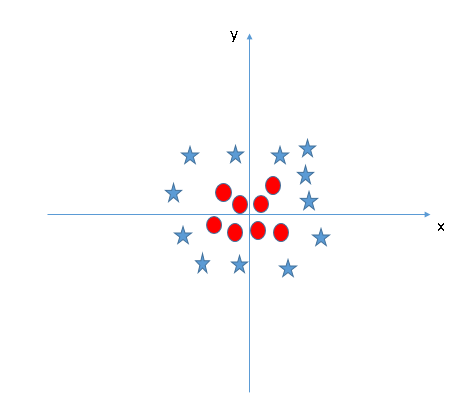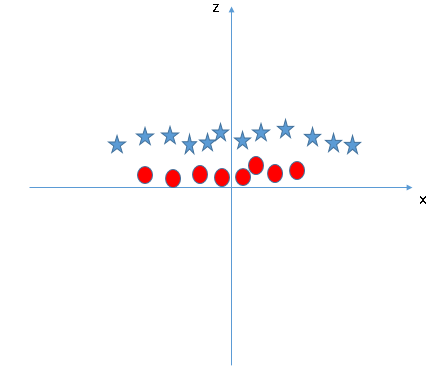ϕ(x⃗ ) ϕ ( x → ) $\phi(\vec{x})$表示将样本点 x⃗  x → $\vec{x}$映射后的特征向量，类似于线性可分支持向量机中的表示方法，在特征空间中划分超平面所对应的模型可表示为
f(x⃗ )=w⃗ Tx+b(25) (25) f ( x → ) = w → T x + b

$\begin{array}{}\text{(26)}& \left\{\begin{array}{l}\underset{\stackrel{\to }{w},b}{min}\frac{1}{2}||\stackrel{\to }{w}|{|}^{2}\\ s.t.\phantom{\rule{1em}{0ex}}{y}_{i}\left({\stackrel{\to }{w}}^{T}\varphi \left(\stackrel{\to }{x}\right)+b\right)\ge 1\phantom{\rule{1em}{0ex}},i=1,2,\dots ,n\end{array}\end{array}$

maxαni=1αi12ni=1nj=1αiαjyiyjϕ(xiT)ϕ(xj)s.t.αi0,i=1,2,,nni=1αiyi=0(27) (27) { max α ∑ i = 1 n α i − 1 2 ∑ i = 1 n ∑ j = 1 n α i α j y i y j ϕ ( x i → T ) ϕ ( x j → ) s . t . α i ≥ 0 , i = 1 , 2 , … , n ∑ i = 1 n α i y i = 0

κ(xi,xj)=ϕ(xiT)ϕ(xj)(28) (28) κ ( x i → , x j → ) = ϕ ( x i → T ) ϕ ( x j → )

maxαni=1αi12ni=1nj=1αiαjyiyjκ(xi,xj)s.t.αi0,i=1,2,,nni=1αiyi=0(29) (29) { max α ∑ i = 1 n α i − 1 2 ∑ i = 1 n ∑ j = 1 n α i α j y i y j κ ( x i → , x j → ) s . t . α i ≥ 0 , i = 1 , 2 , … , n ∑ i = 1 n α i y i = 0

f(x⃗ )=w⃗ Tϕ(x⃗ )+b=i=1nαiyiϕ(x⃗ )Tϕ(x⃗ )+b=i=1nαiyiκ(xi,xj)+b(30) (30) f ( x → ) = w → T ϕ ( x → ) + b = ∑ i = 1 n α i y i ϕ ( x → ) T ϕ ( x → ) + b = ∑ i = 1 n α i y i κ ( x i → , x j → ) + b

## 支持向量回归

minw⃗ ,b(12||w⃗ ||2+Ci=1nLε(f(x⃗ )yi))(31) (31) min w → , b ( 1 2 | | w → | | 2 + C ∑ i = 1 n L ε ( f ( x → ) − y i ) )

Lε(z)={0,|z|ε|z|ξ,otherwise(32) (32) L ε ( z ) = { 0 , | z | ≤ ε | z | − ξ , o t h e r w i s e

minw⃗ ,b,ξ,ξ^i(12||w⃗ ||2+Cni=1(ξi+ξ^i))s.t.f(xi)yiε+ξi,i=1,2,,nyif(x⃗ )ε+ξ^iξi0,ξ^i0(33) (33) { min w → , b , ξ , ξ ^ i ( 1 2 | | w → | | 2 + C ∑ i = 1 n ( ξ i + ξ ^ i ) ) s . t . f ( x i → ) − y i ≤ ε + ξ i , i = 1 , 2 , … , n y i − f ( x → ) ≤ ε + ξ ^ i ξ i ≥ 0 , ξ ^ i ≥ 0

L(w⃗ ,b,α⃗ ,α^⃗ ,ξ⃗ ,ξ^⃗ ,μ⃗ ,μ^⃗ )=12||w⃗ ||2+Ci=1n(ξ+ξ^)i=1nμiξii=1nμ^iξ^i+i=1nαi(f(xi)yiεξ)+i=1nα^i(yif(xi)εξ^i)(34) (34) L ( w → , b , α → , α ^ → , ξ → , ξ ^ → , μ → , μ ^ → ) = 1 2 | | w → | | 2 + C ∑ i = 1 n ( ξ + ξ ^ ) − ∑ i = 1 n μ i ξ i − ∑ i = 1 n μ ^ i ξ ^ i + ∑ i = 1 n α i ( f ( x i → ) − y i − ε − ξ ) + ∑ i = 1 n α ^ i ( y i − f ( x i → ) − ε − ξ ^ i )
L(w⃗ ,b,α⃗ ,α^⃗ ,ξ⃗ ,ξ^⃗ ,μ⃗ ,μ^⃗ ) L ( w → , b , α → , α ^ → , ξ → , ξ ^ → , μ → , μ ^ → ) $L(\vec{w},b,\vec{\alpha},\vec{\hat\alpha},\vec{\xi},\vec{\hat\xi},\vec{\mu},\vec{\hat\mu})$ w⃗ ,b,ξ⃗ ,ξ^⃗  w → , b , ξ → , ξ ^ → $\vec{w},b,\vec{\xi},\vec{\hat\xi}$的偏导数为零可得：
w⃗ =ni=1()xini=1(α^iαi)=0C=αi+μiC=α^i+μ^i(35) (35) { w → = ∑ i = 1 n ( ) x i → ∑ i = 1 n ( α ^ i − α i ) = 0 C = α i + μ i C = α ^ i + μ ^ i

{maxα,α^ni=1(yi(α^iαi)ε(α^i+αi)12n